Standard

# UNIT 1: REAL NUMBERS

Page

## Click here to go to the IXL website for all kinds of 8th grade topics and review problems.

To practice Classifying Real Numbers click here.

# REAL NUMBERS

In this unit we went over Real Numbers and the classification system that is set up with Real Numbers. Here is the Rational Numbers Poster we created in classWe used Nesting boxes to demonstrate how the sub groups are inside each other.

 Description of Each Set of Numbers Natural The natural numbers (also known as counting numbers) are the numbers that we use to count. It starts with 1, followed by 2, then 3, and so on.1, 2, 3, 4… Whole The whole numbers are a slight “upgrade” of the natural numbers because we simply add the number zero to the current set of natural numbers. Think of whole numbers as natural numbers and the number zero.0, 1, 2, 3, 4… **Remember a hole in the ground is shaped like the number zero!** Integers The integers include all whole numbers together with the “opposites” of the natural numbers (their negatives).… -4, -3, -2, -1, 0, 1, 2, 3, 4 … Rational The rational numbers are numbers which can be expressed as ratio of integers. That means, if we can write a given number as a fraction where the numerator and denominator are both integers; then it is a rational number.Caution: The denominator cannot equal to zero. Rational numbers can also appear in decimal form. If the decimal number either terminates or repeats, then it is possible to write it as a fraction with an integer numerator and denominator. Thus, it is rational as well Irrational The irrational numbers are all numbers which when written in decimal form does not repeat and does not terminate. Real The real numbers includes both the rational and irrational numbers. Remember that under the rational number, we have the subcategories of integers, whole numbers and natural numbers.

# Ordering Numbers

Watch a video about ordering number here!

To order numbers there are a couple steps to follow.

1st: Convert all you numbers to decimals

2nd: Convert these to percents

3rd: Label a number line

4th: Put your numbers on the number line

## Ascending Order

To put numbers in order, place them from lowest (first) to highest (last).

This is called “Ascending Order”.

Example: Place 17, 5, 9 and 8 in ascending order.

• Answer: 5, 8, 9, 17

## Descending Order

Sometimes you want the numbers to go the other way, from highest down to lowest, this is called “Descending Order”.

Example: Place 17, 5, 9 and 8 in descending order.

• Answer: 17, 9, 8, 5

#### Click here  or here    or here   or here  or here to practice Ordering Numbers online and get automatic feedback (it grades it)! 🙂# Scientific Notation

Scientific notation is about writing really big and really small numbers in an equivalent form.

We normally write numbers in what we call STANDARD NOTATION, but we can also write this number in SCIENTIFIC NOTATION.

For example the number three hundred and twenty.

STANDARD:   320

SCIENTIFIC NOTATION: 3.2 × 102

## CONVERTING NUMBERS

When given a number in scientific notation you can easily convert the number to standard notation.

Example #1

Convert from Scientific Notation:  3.6 × 1012

to Standard Notation

Now, since the exponent on 10 is positive, I know they are looking for a LARGE number, so I’ll need to move the decimal point in the positive direction (to the right), in order to make the number LARGER. Since the exponent on 10 is “12“, I’ll need to move the decimal point twelve places over.

First, I’ll move the decimal point twelve places over. I make little loops when I count off the places, to keep track: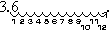Then I fill in the loops with zeroes: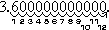In other words, the number is 3,600,000,000,000, or 3.6 trillion

Example #2

Convert 4.2 × 10–7 to standard notation.

Since the exponent on 10 is negative, I am looking for a small number. Since the exponent is a seven, I will be moving the decimal point seven places. Since I need to move the point to get a small number, I’ll be moving it to the left. The answer is 0.00000042

# Wednesday 12 ENRICHMENT

Standard

2nd Period click here to go to Desmos and Play Guess Who with Lines.

6th Period click here to go to Desmos and Play Guess Who with Lines.

# Let’s Play!

Standard

2nd Period click here to go to Desmos to play Guess Who with Points.

6th Period click here to go to Desmos to play Guess Who with Points.

Standard

# REAL NUMBERS

In this unit we went over Real Numbers and the classification system that is set up with Real Numbers. Here is the Rational Numbers Poster we created in classWe used Nesting boxes to demonstrate how the sub groups are inside each other.

 Description of Each Set of Numbers Natural The natural numbers (also known as counting numbers) are the numbers that we use to count. It starts with 1, followed by 2, then 3, and so on. 1, 2, 3, 4… Whole The whole numbers are a slight “upgrade” of the natural numbers because we simply add the number zero to the current set of natural numbers. Think of whole numbers as natural numbers and the number zero. 0, 1, 2, 3, 4… **Remember a hole in the ground is shaped like the number zero!** Integers The integers include all whole numbers together with the “opposites” of the natural numbers (their negatives). … -4, -3, -2, -1, 0, 1, 2, 3, 4 … Rational The rational numbers are numbers which can be expressed as ratio of integers. That means, if we can write a given number as a fraction where the numerator and denominator are both integers; then it is a rational number. Caution: The denominator cannot equal to zero. Rational numbers can also appear in decimal form. If the decimal number either terminates or repeats, then it is possible to write it as a fraction with an integer numerator and denominator. Thus, it is rational as well Irrational The irrational numbers are all numbers which when written in decimal form does not repeat and does not terminate. Real The real numbers includes both the rational and irrational numbers. Remember that under the rational number, we have the subcategories of integers, whole numbers and natural numbers.

# Ordering Numbers

Watch a video about ordering number here!

To order numbers there are a couple steps to follow.

1st: Convert all you numbers to decimals

2nd: Convert these to percents

3rd: Label a number line

4th: Put your numbers on the number line

## Ascending Order

To put numbers in order, place them from lowest (first) to highest (last).

This is called “Ascending Order”.

Example: Place 17, 5, 9 and 8 in ascending order.

• Answer: 5, 8, 9, 17

## Descending Order

Sometimes you want the numbers to go the other way, from highest down to lowest, this is called “Descending Order”.

Example: Place 17, 5, 9 and 8 in descending order.

• Answer: 17, 9, 8, 5

#### Click here  or here    or here   or here  or here to practice Ordering Numbers online and get automatic feedback (it grades it)! 🙂# Scientific Notation

Scientific notation is about writing really big and really small numbers in an equivalent form.

We normally write numbers in what we call STANDARD NOTATION, but we can also write this number in SCIENTIFIC NOTATION.

For example the number three hundred and twenty.

STANDARD:   320

SCIENTIFIC NOTATION: 3.2 × 102

## CONVERTING NUMBERS

When given a number in scientific notation you can easily convert the number to standard notation.

Example #1

Convert from Scientific Notation:  3.6 × 1012

to Standard Notation

Now, since the exponent on 10 is positive, I know they are looking for a LARGE number, so I’ll need to move the decimal point in the positive direction (to the right), in order to make the number LARGER. Since the exponent on 10 is “12“, I’ll need to move the decimal point twelve places over.

First, I’ll move the decimal point twelve places over. I make little loops when I count off the places, to keep track:Then I fill in the loops with zeroes:In other words, the number is 3,600,000,000,000, or 3.6 trillion

Example #2

Convert 4.2 × 10–7 to standard notation.

Since the exponent on 10 is negative, I am looking for a small number. Since the exponent is a seven, I will be moving the decimal point seven places. Since I need to move the point to get a small number, I’ll be moving it to the left. The answer is 0.00000042

#### Click here to do Scientific Notation practice online and get automatic feedback (it grades it)! 🙂Standard

# SLOPE = m

## Steps to calculate slope:

1. In order to calculate slope you need……. 2 PERFECT POINTS.  Write them down.
2. Once you have the 2 points/ordered pairs you can find the slope formula on the…… STAAR CHART. Write the formula down.
3. Now you MUST LABEL your 2 perfect points. ***Remember it is (x, y) the x value is 1st
4. Now that your points are labeled you can PLUG IN or substitute your numbers in the formula.
5. You can solve using your…… CALCULATOR.
6. If you get a decimal and want a fraction use your calculator and push the MATH button then ENTER and ENTER again and your answer should be in fractions form.

## Steps to calculate slope using only the graphing calculatorOther things you should know about SLOPE:                                                                                      Slope is a RATE, that means it happens again and again and again (multiple times, M). It is the ratio that explains how steep a line is. When you think of steepness think of a hill. The steeper the hill the harder it would be to walk up it. The harder to walk up the hill the greater the slope.

### Example: The RED line is steeper than the blue line. It would be harder to walk on. If you look at the  y=   equations on the right you can also see the slope of the RED line is greater than the slope of the BLUE line.– Love Ya’ll!!! Mrs. Molina 🙂

# Y-Intercept = b

## Steps to find the y-intercept:

2. Notice where the lines touches or meets the y-axis ( the one that goes up and down)
3. The y value at this location is the y- intercept.

Example: The y-intercept (b) = 1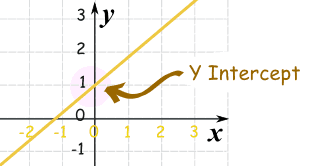# Unit Rate and Slope

Unit Rate and Slope are almost the EXACT SAME THING.

The ONLY difference is that Slope is just a number and Unit Rate must have a description with it.

Example:

# PYTHAGOREAN THEOREM

The Pythagorean Theorem states that, in a right triangle, the two smaller squares made from the triangles legs add up to equal the biggest square made from the hypotenuse.The lengths of the legs are commonly labeled as a and b and the hypotenuse length is labeled as c.REMEMBER:

HYPOTENUSE: c is the longest side of the triangle

LEGS: a and b are the other two sidesClick here  to find the missing hypotenuse , to find the missing leg, here  to find the perimeter, here  to do real world problems, and here to see if the triangle is a right triangle or not. You will use the Pythagorean Theorem online and get automatic feedback (it grades it)! 🙂

## What is the Pythagorean Theorem?

In short the Pythagorean Theorem can be explain with the formula below.

## a2 + b2 = c2

AGAIN, THIS IS ONLY TRUE FOR RIGHT TRIANGLES!

Here are other examples: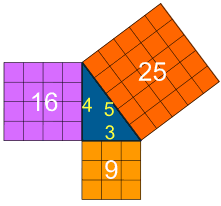Click here  to find the missing hypotenuse , to find the missing leg, here  to find the perimeter, here  to do real world problems, and here to see if the triangle is a right triangle or not. You will use the Pythagorean Theorem online and get automatic feedback (it grades it)! 🙂

Standard

# Volume

Volume is the measure of the inside of a 3-D figure.

The amount of 3-dimensional space an object occupies. Capacity.The formula is V=Bh

B=Area of the Base (use the STAAR Chart to find B)
h=height of Prism

Units of volume include:
Metric: cubic centimeters (cm3), cubic meters (m3)In this 3-D figure:

B= bh = 10(5) =50

h= 4

So, V= (550)(4) = 200 units³

For this example the volume is 200 units3

Practice Volume here and here .

# Surface Area

The total area of the surface of a three-dimensional object.

Example: the surface area of a cube is the area of all 6 faces added together.
The formula is S = Ph + 2B

P=Perimeter of the Base (add all the sides of the base together)
h=height of Prism
B=Area of the Base (use the STAAR Chart to find B)
Units of volume include:
Metric: cubic centimeters (cm2), cubic meters (m2),

Practice Surface Area here.

Standard

# PYTHAGOREAN THEOREM

The Pythagorean Theorem states that, in a right triangle, the two smaller squares made from the triangles legs add up to equal the biggest square made from the hypotenuse.The lengths of the legs are commonly labeled as a and b and the hypotenuse length is labeled as c.REMEMBER:

HYPOTENUSE: c is the longest side of the triangle

LEGS: a and b are the other two sidesClick here  to find the missing hypotenuse , to find the missing leg, here  to find the perimeterhere  to do real world problems, and here to see if the triangle is a right triangle or not. You will use the Pythagorean Theorem online and get automatic feedback (it grades it)! 🙂

## What is the Pythagorean Theorem?

In short the Pythagorean Theorem can be explain with the formula below.

## a2 + b2 = c2

AGAIN, THIS IS ONLY TRUE FOR RIGHT TRIANGLES!

Here are other examples:Click here  to find the missing hypotenuse , here  to find the missing leg, here  to find the perimeter, here  to do real world problems, and here to see if the triangle is a right triangle or not. You will use the Pythagorean Theorem online and get automatic feedback (it grades it)! 🙂

# ANGLE and TRIANGLE RELATIONSHIPS

## Degrees

A degree is a unit of measurement used to measure angles.

### We can measure all Angles in Degrees.

There are 360 degrees in one Full Rotation (one
complete circle around

## The Degree Symbol: °

We use a little circle ° following the number to mean degrees.

For example 90° means 90 degrees

## Angles### In One Diagram

This diagram might make it easier to remember:

Also: Acute, Obtuse and Reflex are in alphabetical order.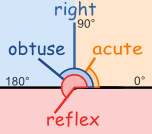# Supplementary Angles

Two Angles are Supplementary if they add up to 180 degrees.
 These two angles (140° and 40°) are Supplementary Angles, because they add up to 180°.Notice that together they make astraight angle.But the angles don’t have to be together.These two are supplementary because 60° + 120° = 180°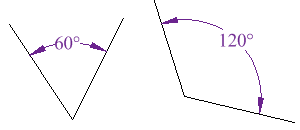# Complementary Angles

Two angles are Complementary when they
(a Right Angle).

 These two angles (40° and 50°) are Complementary Angles, because they add up to 90°.Notice that together they make a right angle.But the angles don’t have to be together.These two are complementary because 27° + 63° = 90°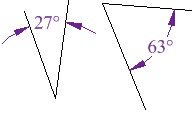# Angle Relationships

Click here, here, and here to find the missing angles of triangles and parallel lines cut by a transversal online and get automatic feedback (it grades it)! 🙂

# Interior Angle

### An Interior Angle is an angle inside a shape.# Exterior Angle

### The Exterior Angle is the angle between any side of a shape, and a line extended from the next side.# Angles On One Side of A Straight Line

### Angles on one side of a straight line will always add to 180 degrees.

If a line is split into 2 and you know one angle you can always find the other one.30° + 150° = 180°

# Interior Angles of Polygons

### An Interior Angle is an angle inside a shape.## Triangles

The Interior Angles of a Triangle add up to 180°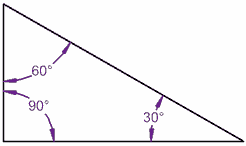### 80° + 70° + 30° = 180°

It works for this triangle!

Let’s tilt a line by 10° …

It still works, because one angle went up by 10°, but the other went down by 10°

(A Quadrilateral has 4 straight sides)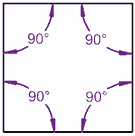### 80° + 100° + 90° + 90° = 360°

A Square adds up to 360°

Let’s tilt a line by 10° … still adds up to 360°!

## Because there are Two Triangles in a Square

 The interior angles in this triangle add up to 180°(90°+45°+45°=180°)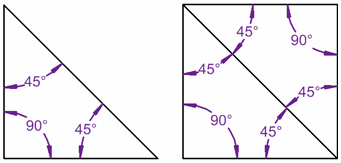Standard

# TRANSFORAMTIONS

There are 4 types of TRANSFORMATIONS that we will be learning about.Click here to view information on all 4 transformations (translations, reflections, rotations, and dilations) or click here to play a video that explain each transformation.

Click here or here or here to practice identifying the Transformation that is taking place and get automatic feedback (it grades it)! 🙂

##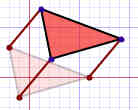Click here  or here  or here to practice Translations and get automatic feedback (it grades it)! 🙂##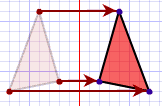Click here or here or here to practice Reflections and get automatic feedback (it grades it)! 🙂##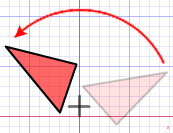Click here or here or here to practice Rotations and get automatic feedback (it grades it)! 🙂# How do I know if the shape is Congruent or Similar?

If one shape can become another using only Turns, Flips and/or Slides, then the two shapes are called Congruent.

Two shapes are Similar if you need to Resize for one shape to become another (you may also Turn, Flip and/or Slide).

## Dilation –> Enlarge/Grow or Reduce/Shrink

The other important Transformation is Dilation (also called reduction or enlargement). The new image becomes bigger or smaller than the original.Click here or here or here  or here to practice Dilations and get automatic feedback (it grades it)! 🙂

### Click here for a vocabulary list or here for flashcard practice.

Standard

#### The graphs are about having data for two variables (x, y) . Typically the two types of data are related in some way.

Below is a Scatter Plot with bivariate data. The two things that we are comparing are Temperature and Ice Cream Sales.

Here we have ice cream sales versus the temperature on that day. The two variables are Ice Cream Sales and Temperature.

###### (If you have only one set of data, such as just Temperature, it is called “Univariate Data, bivariate mean two types of data)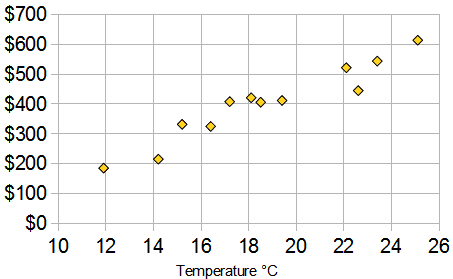In this scatter plot you can see that as tempurate rises so do prices.

https://www.mathsisfun.com/definitions/bivariate-data.html

Click here and  here   and here and here to practice Identifying Scatter Plot Trends and Predicting with Best Fit Lines online and get automatic feedback (it grades it)! 🙂

## Writing Linear Equations

### Slope Intercept Form:      y = mx + b

The variable m is the slope, it explains the steepness of a line.

The variable b is the y-intercept, this is where the line touches or intersects at the y-axis.

http://www.shmoop.com/video/slope-intercept-form/

Standard